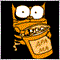# Loop to find daily price range of last x day72

Hi, how can I loop through days to find minvalue and max value, it would be interesting to write to file the following informations:

<Day number>,<session>,<MinValInRange>,<MaxValInRange>,<NumPipsInRange>,<VarPercent>

Variables:

`string Fname = Symbol()+".csv";//filename with current symbol`

The first 2 functions could be:

```double MaxVal(datetime t1, datetime t2)
{
string   s           = Symbol();
int      p           = PERIOD_H1;
int iHiH1;
double HighestV;
int      t1_shift    = iBarShift(s,p,t1);
int      t2_shift    = iBarShift(s,p,t2);
int      bar_count   = t1_shift-t2_shift;
iHiH1    = iHighest( s, p, MODE_HIGH,bar_count,t2_shift);
HighestV = iHigh(s, p, iHiH1);
return HighestV;
}```

and

```double MinVal(datetime t1, datetime t2)
{
string   s           = Symbol();
int      p           = PERIOD_H1;
int iLoH1;
double LowestV;
int      t1_shift    = iBarShift(s,p,t1);
int      t2_shift    = iBarShift(s,p,t2);
int      bar_count   = t1_shift-t2_shift;
iLoH1    = iLowest(s, p, MODE_LOW,bar_count, t2_shift);
LowestV = iLow(s, p, iLoH1);
return LowestV;
}
```15884

Use loop. Nothing else comes to mind.

```DateTime TMStart;
DateTime TMEnd;
int DayCount   = 5;
int StartIndex = 0;
int EndIndex = 1000;

while (StartIndex<EndIndex)
{
TMStart = Time[StartIndex];
TMEnd   = TMStart + DayCount*24*3600;
MaxVal(TMStart,TMEnd);
StartIndex+=DayCount;
}
```

Some way to loop.72

Vitalii Ananev:

Use loop. Nothing else comes to mind.

Some way to loop.

Time is a (last) candle time, if loops starts from the beginning the first date  should be Time[TMEnd]?15884

andy60:

Time is a (last) candle time, if loops starts from the beginning the first date should be Time[TMEnd]?

Time - this is a current candle not yet closed. If you build calculations from the past to the future. Then you need to change the algorithm a little. In my example, the calculation goes from the current candle to the past.

```DateTime TMStart;
DateTime TMEnd;
int DayCount   = 5;
int StartIndex = 1000;
int EndIndex = 0;

while (StartIndex>EndIndex)
{
TMStart = Time[StartIndex];
TMEnd   = TMStart + DayCount*24*3600;//Date forward to future
MaxVal(TMStart,TMEnd);
StartIndex-=DayCount;
}```

Like this.

In the previous example I was a little wrong I should have written like this:

```DateTime TMStart;
DateTime TMEnd;
int DayCount   = 5;
int StartIndex = 0;
int EndIndex = 1000;

while (StartIndex<EndIndex)
{
TMStart = Time[StartIndex];
TMEnd   = TMStart - DayCount*24*3600;//Date back to the past
MaxVal(TMStart,TMEnd);
StartIndex+=DayCount;
}```72

Vitalii Ananev:

Time - this is a current candle not yet closed. If you build calculations from the past to the future. Then you need to change the algorithm a little. In my example, the calculation goes from the current candle to the past.

Like this.

Thx ! I will try the first solution, i had read bad first expression, it seems perfect.

`StartIndex<EndIndex`15884

andy60:

Thx ! I will try the first solution, i had read bad first expression, it seems perfect.

Give it a try. Maybe someone will offer an even better option. In any case, you must use a loop to calculate MaxVal() and MinVal() each time passing a new date range in the parameters.